Latest Banking jobs   »

# Quantitative Aptitude Quiz For IBPS RRB PO/Clerk Prelims 2022- 14th July

Q1. Akash invests certain sum into two schemes A & B in the ratio of 3: 4 for 3 years. Scheme A and B offered S.I. at the rate of 6% and 12% per annum respectively. If total S.I. obtained from both schemes after 3 years is Rs.3960, then find amount invested by Akash in scheme A?
(a) Rs. 6000
(b) Rs. 5000
(c) Rs. 7500
(d) Rs. 8000
(e) Rs. 2500

Quantitative Quiz For IBPS RRB PO/Clerk Prelims 2022 14th July IN HINDI

Q2. Three partners A, B and C started a business with their initial investments of Rs.6600, Rs.7200 and Rs.9000 respectively. After 4 months, B withdrew Rs.1200 and A invests Rs.600 extra. After a year, if C got Rs.5625 as his profit then find total profit?
(a) Rs. 15,000
(b) Rs. 12,000
(c) Rs. 14,000
(d) Rs. 10,000
(e) Rs. 16,000

Q3. A person purchases bicycle at a discount of 20% on marked price. If sum of discount offered and profit obtained on bicycle is Rs. 480, then find the difference in marked price and cost price on bicycle? (in Rs.)
(a) 520
(b) 480
(c) 122
(d) 880
(e) 690

Q4. A sum on simple interest becomes 5/2 times of itself in ten years, find the rate of interest?
(a) 20%
(b) 10%
(c) 30%
(d) 15%
(e) 12%

Q5. A started a business with investment Rs.2500 and after 6 months of starting the business B also joined with Rs.4000. At the end of year annual profit of business was Rs.3600. Find the profit share of B?
(a) Rs. 1600
(b) Rs. 2000
(c) Rs. 2800
(d) Rs. 3600
(e) None of these

Q6. If the manufacturer gains 20%, the whole-sale dealer 10% and the retailer 25%, then find the cost of production of a table (in Rs.), if the retail price of which is Rs.3300?
(a) Rs. 1400
(b) Rs. 2000
(c) Rs. 1000
(d) Rs. 1200
(e) Rs. 900

Q7. If the rate of interest is 20% p.a. then find the compound interest earned on Rs.1600 in 1 ½ years. If interest is charged half yearly.
(a) Rs. 500.5
(b) Rs. 961.3
(c) Rs. 529.6
(d) Rs. 463.5
(e) Rs. 460.4

Q8. The compound interest and simple interest on a certain sum for two years are Rs.1267.5 and Rs.1200 respectively. If rate of interest for both S.I. and C.I is 15% p.a., then find the sum.
(a) Rs.3000
(b) Rs.2500
(c) Rs.4000
(d) Rs.1800
(e) Rs.2200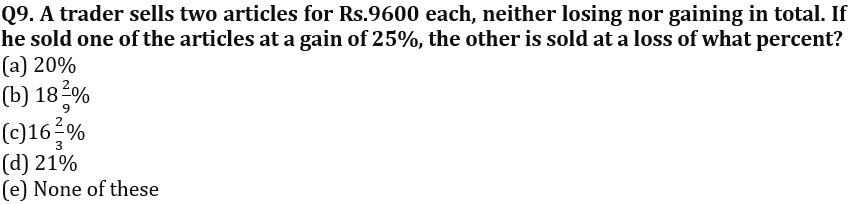Q10. N and M started a business by making investment in the ratio of 3: 4. After four months, M withdraws one fourth of his initial investment. If total annual profit was Rs. 760, find profit share of N?
(a) Rs. 480
(b) Rs. 360
(c) Rs. 420
(d) Rs. 520
(e) Rs. 320

Q11. Raman borrows Rs.12600 from a bank on compound interest at the rate of 33⅓% p.a. for two years. Find the total interest given by Raman to the bank after 2 years?
(a) Rs.7200
(b) Rs.8800
(c) Rs.9800
(d) Rs.4200
(e) Rs. 5600

Q12. Naresh and Ritik enter into a partnership with their initial sum of Rs.18000 and Rs.24000 respectively. After 6 months, a third person Kohli also joins them with his initial sum of Rs.12000. After a year if total profit is Rs. 3200, then find the profit share of Ritik?
(a) Rs. 1500
(b) Rs. 2300
(c) Rs. 1600
(d) Rs. 2800
(e) Rs. 1800

Q13. Two partners A and B enter into a partnership with their initial sum of Rs.25,000 and Rs.20,000 respectively. After 8 months, B left the partnership. If total profit after a year is Rs.3450, then find profit share of A?
(a) Rs. 1850
(b) Rs 1500
(c) Rs 2250
(d) Rs 1450
(e) Rs 1250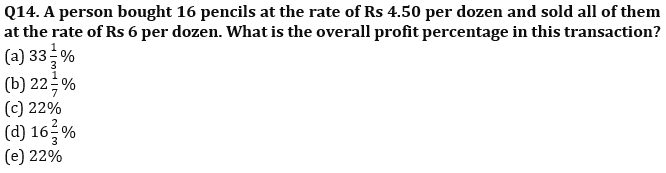Q15. The price of a product after getting 20% discount is Rs.17600 which includes 10% tax on selling price. Find the marked price of the product (in Rs)?
(a) 24000
(b) 18000
(c) 20000
(d) 12000
(e) 30000

Solutions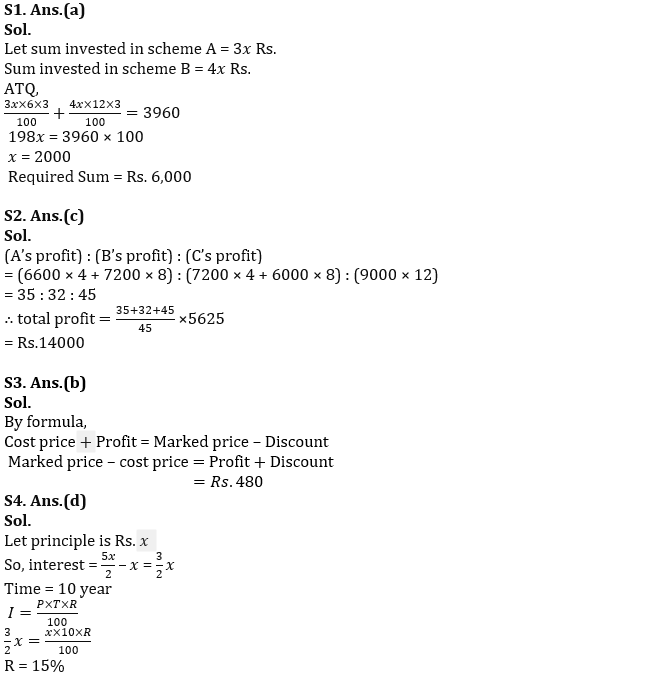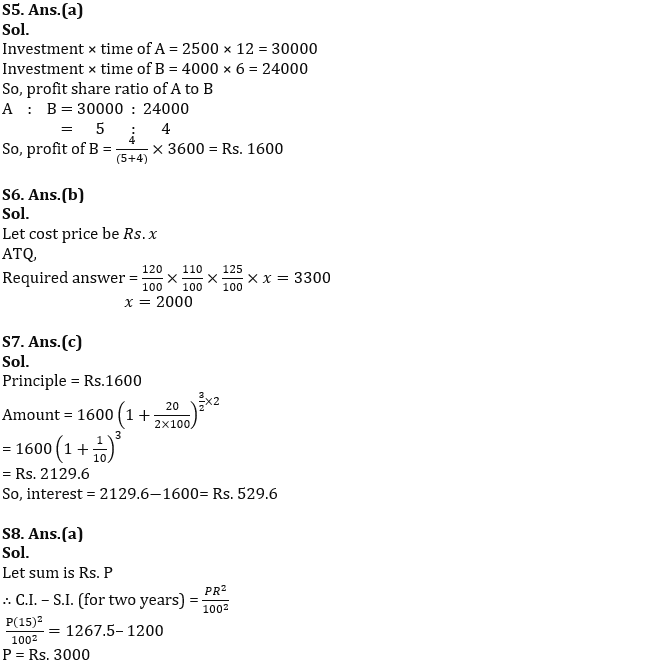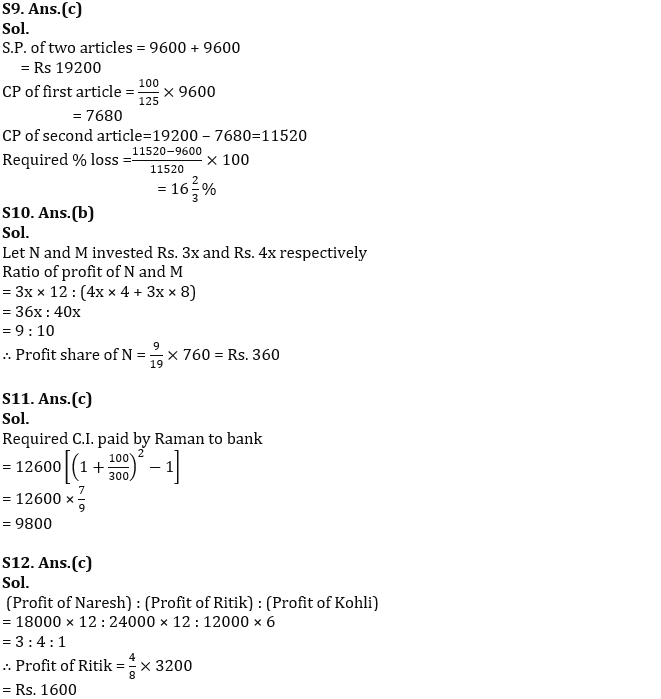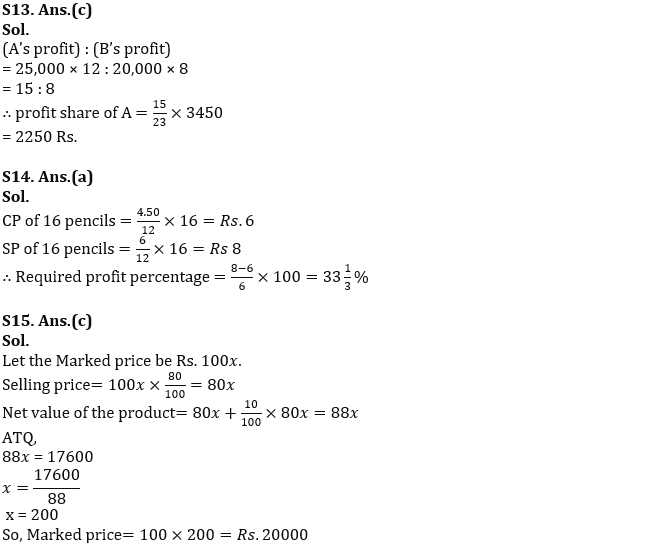#### Congratulations!Incorrect details? Fill the form again here

•Quantitative Aptitude Quiz For IBPS RRB ...
•Reasoning Ability Quiz For IBPS RRB PO C...
•Reasoning Ability Quiz For IBPS RRB PO C...
•Quantitative Aptitude Quiz For IBPS RRB ...
•Reasoning Ability Quiz For IBPS RRB PO C...
•Quantitative Aptitude Quiz For IBPS RRB ...